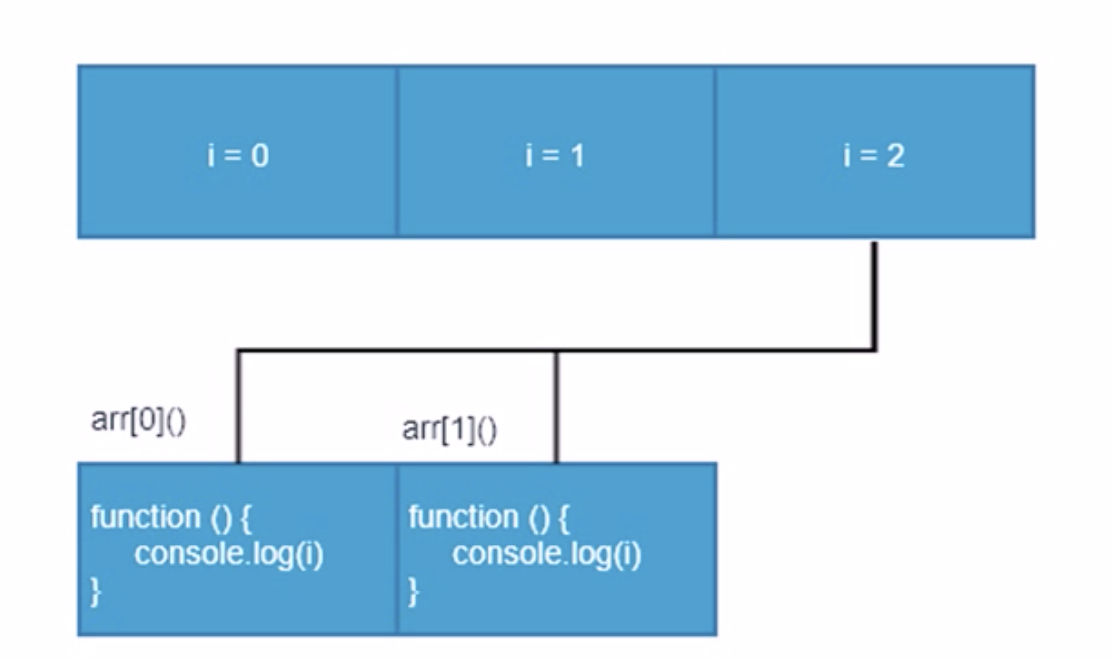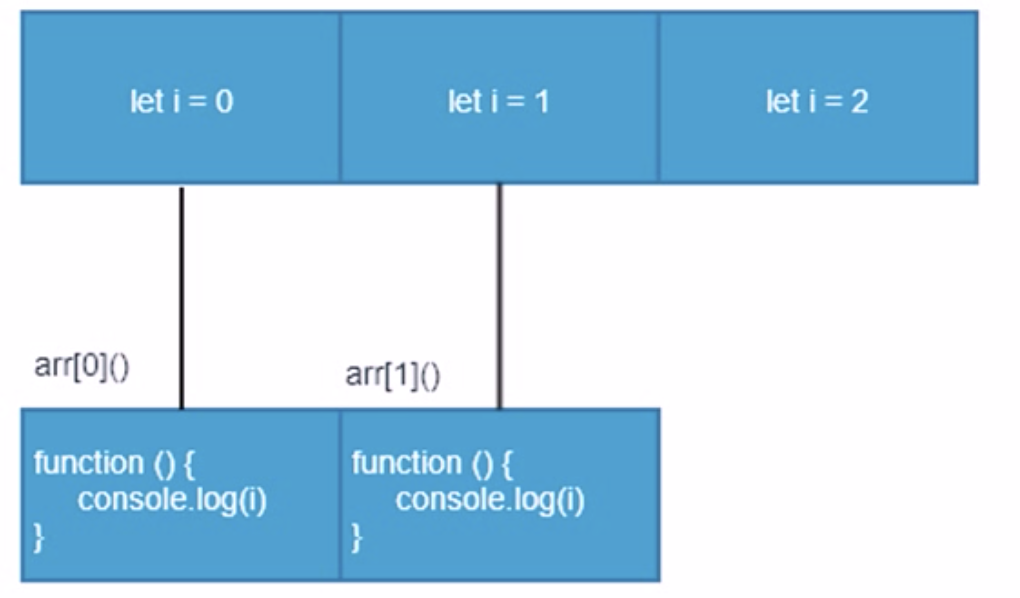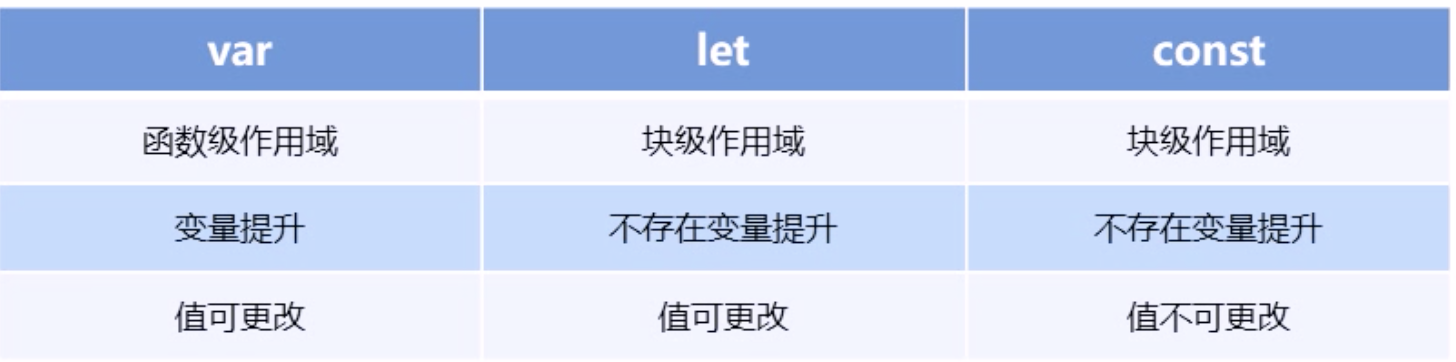# ES6 learning notes (4): easy to understand the new grammar of ES6

2020-11-06 20:42:44

### let

ES6 New keyword used to declare variables

• let Declared variables are only valid at the block level where they are
• No variable promotion
``````//  Use let Declared variables have block level scope
if(true) {

let a = 10
console.log(a) // 10
if(true) {

let c= 30
}
console.log(c) // c is not defined
}
console.log(a) // a is not defined``````

Be careful ： Use let Only variables declared by keyword have block level scope , Use var Declared variables do not have block level scope properties .

In a brace , Use let Only variables declared by keyword have block level scope ,var Keywords don't have this feature .

``````if(true) {

let num = 100
var abc = 200
}
console.log(abc) // 200
console.log(num) // num is not defined``````

Prevent loop variables from becoming global variables

``````for(var i=0; i<2; i++) {

}
console.log(i) //2

for(let j=0; j<2; j++) {

}
console.log(j) //j is not defined``````

Use let Declared variables No variable promotion

``````console.log(a); //a is not defined
let a = 20``````

Use let Declared variables have a temporary dead zone feature

``````var tmp = 123;
if(true) {

tmp = 'abc'
let tmp;   // Cannot access 'tmp' before initialization
}``````

Classic interview example

1. use var Statement
``````var arr = []
for(var i = 0; i<2; i++){

arr[i] = function () {

console.log(i)
}
}
arr(); // 2
arr(); // 2``````

Because of the variable i It has a global effect , So after the final loop, you get i All are 2, After the execution, it is also 2, Here's the picture1. use let Declare variables
``````let arr = []
for(let i = 0; i<2; i++){

arr[i] = function () {

console.log(i)
}
}
arr(); // 0
arr(); // 1``````

Each loop of code produces a block level scope , The variables in each block level scope are different , When the function is executed, the output goes up one level （ Block level scope generated by the loop ） In scope i value### const

effect ： declare constant , A constant is a value （ Memory address ） An unchangeable quantity

• And let equally , Has block level scope
• You must assign a value to a constant when you declare it
• After constant assignment , Value cannot be modified
``````//  Use const  The constant declared by the keyword must be given an initial value
const PI //Missing initializer in const declaration``````

const After the declared constant is assigned , The value cannot be changed any more

``````const PI = 3.14
PI = 100  // Assignment to constant variable.``````

When const When declaring an array of things or objects , Internal values can be changed , But the memory address doesn't change

``````const arr = [100, 200];
arr = 'a';
arr = 'b';
console.log(arr) // ['a', 'b]
arr = ['c','d'] //Assignment to constant variable.``````

### let、const、var The difference between

1. Use var Declared variables , Its scope is within the function of the statement , And there is the phenomenon of variable promotion .
2. Use let Declared variables , Its scope is in the code block where the statement is located , No variable promotion .
3. Use const Declared constant , You can't change the value of this constant in later code .### Deconstruct assignment

ES6 Allows you to extract values from arrays , According to the corresponding position , Assign a value to a variable , Objects can also be deconstructed .

#### An array of deconstruction

Numerical deconstruction allows us to extract values from an array in a one-to-one correspondence and assign values to variables

``````let [a, b, c] = [1, 2, 3]
console.log(a) // 1
console.log(b) //2
console.log(c) //3``````

If deconstruction is not successful , Variable value is undefined

``````let [foo] = [];
let [bar, foo] = 
console.log(bar) //1
console.log(foo) //undefined``````

#### Object to deconstruct

Object deconstruction allows us to use the name of a variable to match the properties of an object , Match successfully assigned the value of the object property to the variable

``````let person = {
name: 'lanfeng', age: 20 }
let {
name, age} = person
console.log(name) //lanfeng
console.log(age) // 20``````

Another way to write object deconstruction

``````let person = {
name: 'lanfeng', age: 20 }
let {
name: myName, age: myAge = 0} = person  //myName,myAge Belong to alias
console.log(myName) //lanfeng
console.log(myAge) // 20``````

### Arrow function

ES6 How to define functions added in the

``````() => {
}
const fn = () => {
}``````

Arrow function is used to simplify the function definition syntax

``````const fn = () => {

console.log(123)
}
fn() //123``````

There is only one code in the function body , And the execution result in the code is the return value , You can omit the braces

``````// ES6 The previous definition method
function sum(num1, num2) {

return num1+ num2
}
const sum = (num1, num2) => num1 + num2``````

If there is only one parameter , You can omit parentheses

``````function fn(v) {

return v
}
const fn v => v``````

Arrow functions are not bound this keyword , In the arrow function this, It points to the context where the function is defined this

``````// Arrow functions are not bound this, Arrow function does not have its own this keyword ,
If you use... In the arrow function this,this The keyword will point to... In the location of the arrow function definition this
const obj = {
name: 'lanfeng'}
function fn() {

console.log(this)
return () => {

console.log(this)
}
}
const resFn = fn.call(obj) //  Point to obj
resFn() //this Point to obj``````

Classic examples of arrow functions ：

``````var obj = {

age: 20,
say: () => {

console.log(this.age)
}
}
obj.say() //undefined, Because it points to window``````

### The remaining parameters

Residual parameter syntax allows us to represent an indefinite number of parameters as an array ,

``````function sum(first, ...args) {

console.log(first) // 10
console.log(args) //[20, 30]
}
sum(10, 20, 30)``````

The remaining parameters are used in conjunction with the deconstruction

``````let arr = ['lanfeg','qianduan', 'yanfa']
let [s1, ...s2] = arr
console.log(s1) //lanfeg
console.log(s2) // ['qianduan', 'yanfa']``````

### summary

This article mainly shares ES6 Some new syntax , such as let、const Declare variable constants , Deconstruct assignment 、 Arrow function 、 Residual function and their respective usage and characteristics .